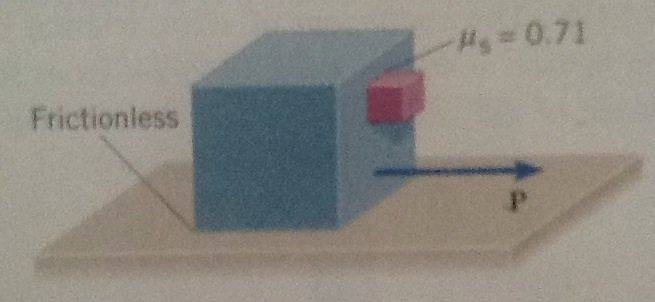# Forces and Newton's Laws of Motion

## Homework Statement

The drawing shows a large cube (mass = 25 kg) being accelerated across a horizontal frictionless surface by a horizontal force P. A small cube (msmall = 4 kg) is in contact with the front surface of the large cube and will slide downward unless P is sufficiently large. The coefficient of friction between the cubes is f = 0.71 . What is the smallest magnitude that P can have in order to keep the small cube from sliding down?Ffriction= f*mg

## The Attempt at a Solution

Ffriction=msmall*g
f*P=msmall*g
P=56,34N

But the answer must be P = 400 N.

mfb
Mentor
P has to accelerate both the small and the large block, that will take more force than the force between the two blocks.

Great answer but still not clear.

Doc Al
Mentor
Ffriction=msmall*g
f*P=msmall*g
P=56,34N
What you found is the force that must push against the small mass, not P, which is the force that pulls the large mass. Use what you found to figure out what P must be.

I kinda figured that out. Can somebody show me how to do it?

Doc Al
Mentor
Here's a hint: Consider the acceleration.

You mean P = ma ?

Doc Al
Mentor
You mean P = ma ?
Yes. Well, F = ma. Consider the acceleration of the small mass first.

The acceleration of the small mass is equal to the large mass.

Doc Al
Mentor
The acceleration of the small mass is equal to the large mass.
Exactly! What's the acceleration of the small mass?

The acceleration of the small mass a = P/msmall

Got it. asmall = 14 m/s2

Plarge = (m + msmall)*a = 400 N

Doc Al
Mentor
The acceleration of the small mass a = P/msmall
No. It's the force acting on the small mass divided by the small mass.

Doc Al
Mentor
Got it. asmall = 14 m/s2

Plarge = (m + msmall)*a = 400 N
Good!